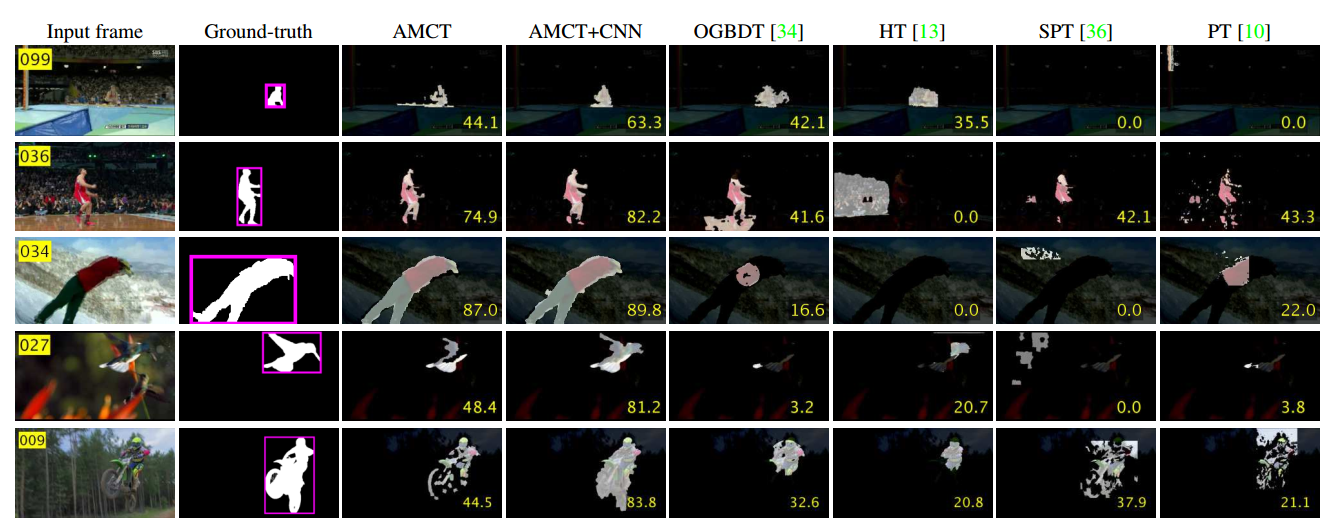# 论文阅读——目标跟踪 2017：Superpixel-based Tracking-by-Segmentation using Markov Chains

### 论文阅读——目标跟踪 2017：Superpixel-based Tracking-by-Segmentation using Markov Chains

• 前言
• 摘要
• 介绍
• 吸收马尔科夫链
• 算法overview
• AMC图的构建
• 使用AMC进行分割跟踪
• 实验部分
• 个人总结

# 前言

## 介绍

1. Wang等人通过meanshift 聚类使用超像素对判断外观进行建模，使用粒子滤波计算最优目标状态。
2. 动态贝叶斯网络跟踪算法采用一个超像素的一组模型来解决非刚性变形问题，而不是用一个单一的整体模型来表示每个目标。

1. Hong 等人提出一种基于使用多个量化级别的分层外观表示的跟踪方法，比如像素，超像素和bounding box.
2. Xiao等人也提出了一个动态多机外观建模的跟踪算法，使用从三个不同级别（像素、超像素和bounding box）获得的信息来维护自适应聚类决策树。

2. 提出的算法根据SVR的得分能够精确地辨别出前景和背景的超像素，与度量学习相比SVR能够更有效的学习鉴别特征。

3. 我们的算法在挑战非刚性和可变形目标跟踪的基准数据集方面明显优于现有技术。

## 吸收马尔科夫链

P=(QR0I)P = \left(\begin{array}{cc} Q &amp; R \\ 0 &amp;I \end{array}\right)

F=∑T=0∞QT=(I−Q)−1F = \sum_{T = 0}^\infty Q^T = (I - Q)^{-1}

## 算法overview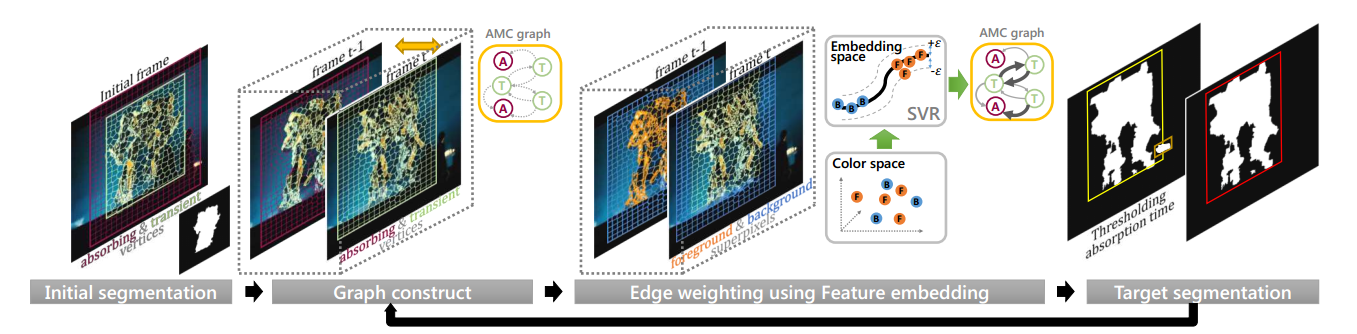## AMC图的构建

{(x1,y1),(x2,y2),...,(xn,yn)}\{(x_1,y_1),(x_2,y_2),...,(x_n,y_n)\}为训练集，前景背景的标签yiy_i分别为1,−11,-1。使用每个超像素块在LAB空间的像素平均值作为超像素的特征，来进行SVR学习。可选地，CNN的特征描述子也可以用于SVR学习。

argminw,ξ,ξ^12∣∣w∣∣2+C∑i=1n(ξi+ξ^i)\mathop{argmin}\limits_{w, \xi, \hat{\xi}} \dfrac{1}{2}||w||^2+C\sum_{i=1}^{n}(\xi_i+\hat{\xi}_i)

yi−&lt;w,Φ(xi)&gt;−b⩽ϵ+ξi,ξi⩾0y_i - &lt;w,\Phi(x_i)&gt; - b \leqslant \epsilon + \xi_i, \xi_i\geqslant 0
&lt;w,Φ(xi)&gt;+b−yi⩽ϵ+ξi^,ξi^⩾0&lt;w,\Phi(x_i)&gt; + b - y_i \leqslant \epsilon + \hat{\xi_i}, \hat{\xi_i}\geqslant 0

κ(xi,xj)=&lt;Φ(xi),Φ(xj)&gt;=exp(∣∣xi−xj∣∣2γ2)\kappa(x_i,x_j)=&lt;\Phi(x_i), \Phi(x_j)&gt; = exp\left(\dfrac{||x_i-x_j||^2}{\gamma^2}\right)

ri=f(xi)=&lt;w,Φ(xi)&gt;r_i = f(x_i) = &lt;w, \Phi(x_i)&gt;

wij=exp(−∣σi−σj∣σr)w_{ij} = exp\left(-\dfrac{|\sigma_i-\sigma_j|}{\sigma_r}\right)

## 使用AMC进行分割跟踪

qij=πtwij∑l=1∣V∣πilwil,rik=πawik∑l=1∣V∣πilwilq_{ij}=\dfrac{\pi_tw_{ij}}{\sum_{l=1}^{|V|}\pi_{il}w_{il}},r_{ik}=\dfrac{\pi_aw_{ik}}{\sum_{l=1}^{|V|}\pi_{il}w_{il}}

πil={πtifvl∈VTπaifvl∈VA\pi_{il}=\left\{\begin{array}{ll}\pi_t &amp; if~v_l \in V^T\\ \pi_a &amp; if ~ v_l \in V^A\end{array}\right.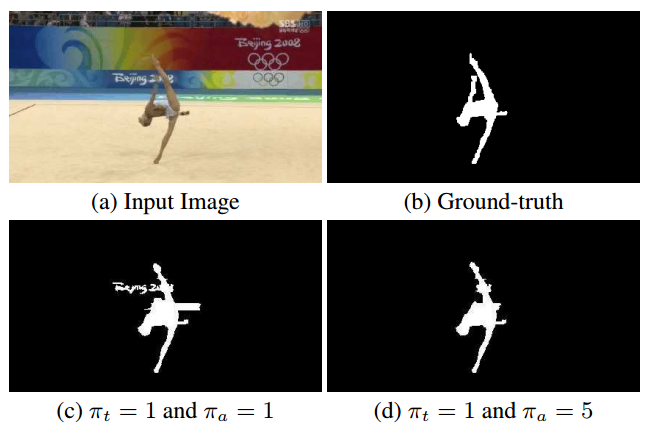yinew=∑vj∈Vt−1Ffijy_i^{new} = \sum_{v_j\in V^{F}_{t-1}}f_{ij}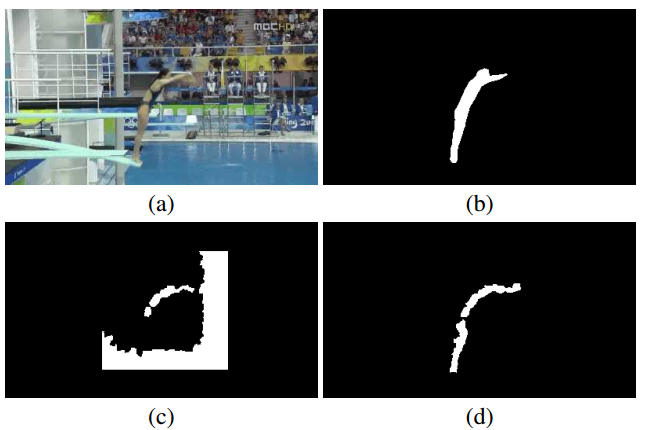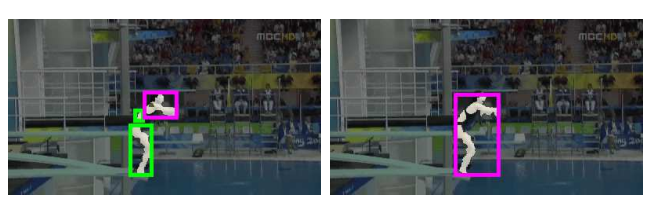htnew=(1−wc)⋅ht+wc⋅hch_t^{new} = (1-w_c)\cdot h_t + w_c\cdot h_c

wij0=exp(−∣∣ci−cj∣∣σc)w^0_{ij} = exp\left(-\dfrac{||c_i-c_j||}{\sigma_c}\right)

## 实验部分